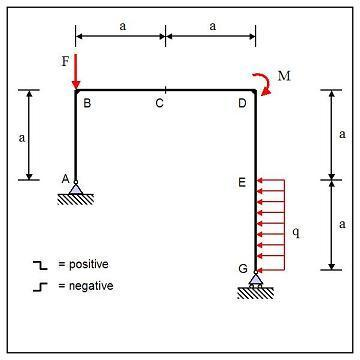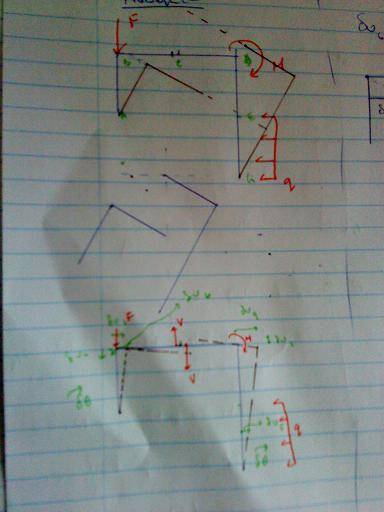# Statics 2D Virtual Work problem regarding calculation of Normal force

manan1
PROBLEM 1

## Homework StatementThe frame in the figure is supported by a hinge in A and a roller in G. It is loaded by a couple = 14 kN*m in B, a force = 12 kN in D and a distributed force = 4 kN/m on section EG. = 1.4 m.
Calculate the normal force in C. Use the correct signs for tension and compression. Hint: Solve using the principle of virtual work and use previously mastered methods to check your answer.

This is a problem from mastering engineering, the statics book. I can solve the problem using equilibrium equations, but i want to know what i am doing wrong with when I am doing using the virtual work theorem.

## Homework Equations

$\delta$W = 0
$\delta\theta small → tan ( \delta\theta) ≈ \delta\theta ;$

## The Attempt at a Solution$\delta\theta_{1} = \delta\theta_{2} = \delta\theta$

$\delta\theta_{1} = \delta u_{1}/a$
$\delta\theta_{2} = \delta u_{2}/2a$

LET
$\delta u_{2} = \delta u$

$\delta u_{2}/2a = \delta u_{1}/a → \delta u_{1} = \delta u/2$
$\delta u_{3}/(a/2) = \delta u/2a → \delta u_{3} = \delta u/4$
$\delta u_{5} = \delta u_{1} = \delta u/2$
$\delta u_{4} = \delta u_{2} = \delta u$

$\delta W = 0$

Therefore
$-M*\delta\theta-N*\delta u_{5}-N*\delta u_{4}-F*\delta u_{2}-qa*\delta u_{3} = 0$
$\delta u ≠ 0$
$-M*\delta u/2a-N*\delta u/2-N*\delta u-F*\delta u-qa*\delta u/4 = 0$
$-\frac{3}{2}N = M/2a + F + qa/4$
N = -12.2666... kN

But the answer should be -17.6 kN according to equilibrium equations.

PROBLEM 2

## Homework StatementThe frame in the figure is supported by a hinge in A and a roller in G. It is loaded by a couple = 4 kN*m in D, a force = 6 kN in B and a distributed force = 1 kN/m on section EG. = 1.5 m
Calculate the shear force in C with the sign convention as shown in the figure. Hint: Solve using the principle of virtual work and use previously mastered methods to check your answer.

## Homework Equations

$\delta$W = 0
$\delta\theta small → tan ( \delta\theta) ≈ \delta\theta ;$

## The Attempt at a SolutionI have no idea how to calculate the virtual displacement for the force F at B.

#### Attachments

Last edited:

piewyz
For problem 1, why are you sure that δθ1=δθ2 ?

manan1
i induce the same amount of rotation in both, and than follow to measure the change in the distances

piewyz
I would try the other way around : assuming a deplacement δu2=δu1 and then calculate the rotation

manan1
tried it, doesnt work.

the new virtual work equation becomes...

-M/a - F - qa/4 = 2N
=> N = -11.7

Last edited: